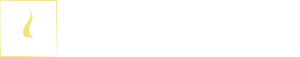# Band-Pass Filters (BPF) - Active Band Pass Filter and Passive Band Pass Filter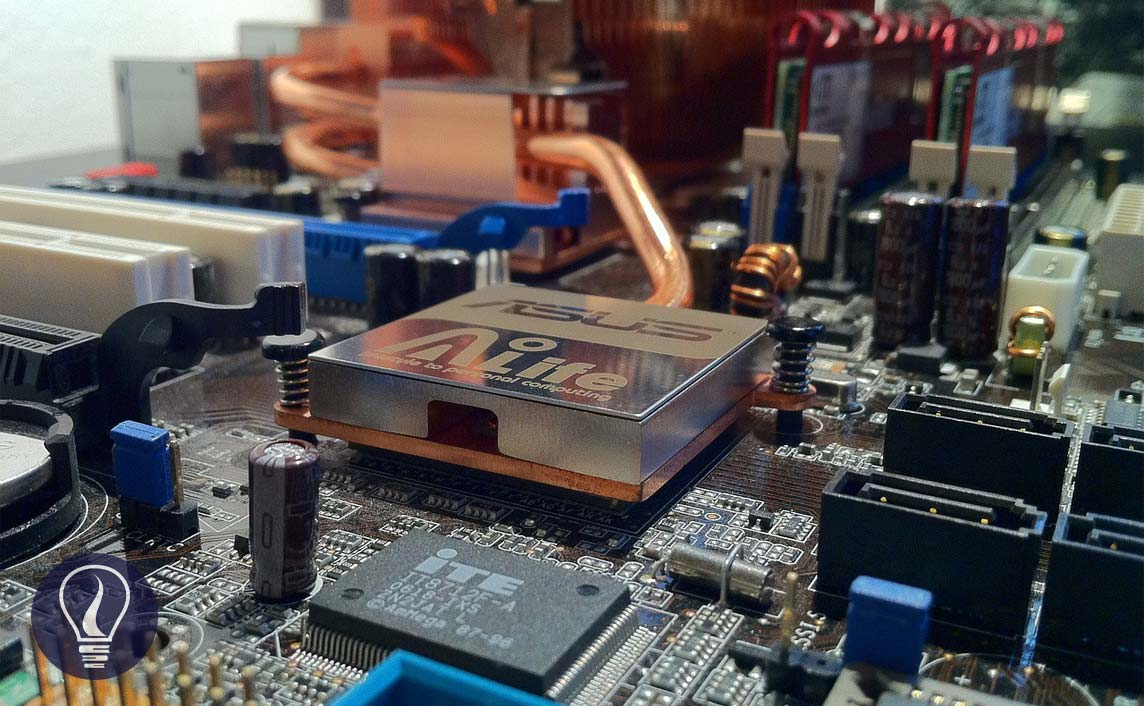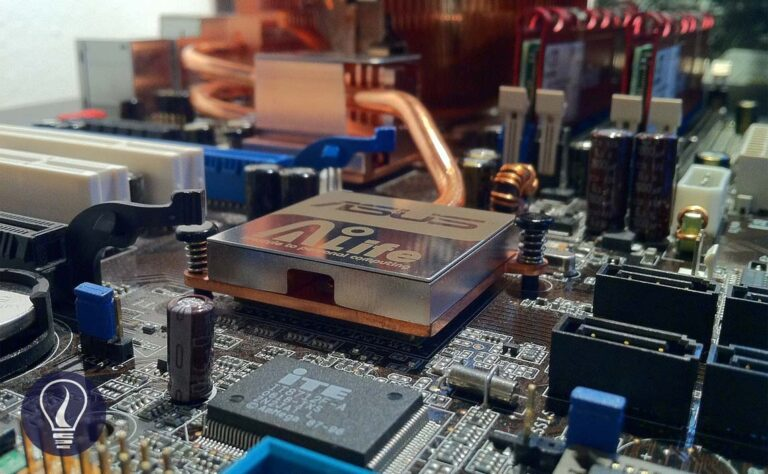B.Sc (Hons, USJ) (Polymer Science and Technology, Chemistry, Physics)
Categories
Tags :
Last Updated On : August 13, 2022
Published Date : August 13, 2022

A band-pass filter is an electronic filter that passes all signals lying within a band between a lower-frequency limit and an upper-frequency limit. So, the band pass filter (BPF) will reject all other frequencies that are outside above specified band. The band-pass filter is a combination of a high pass filter and a low pass filter.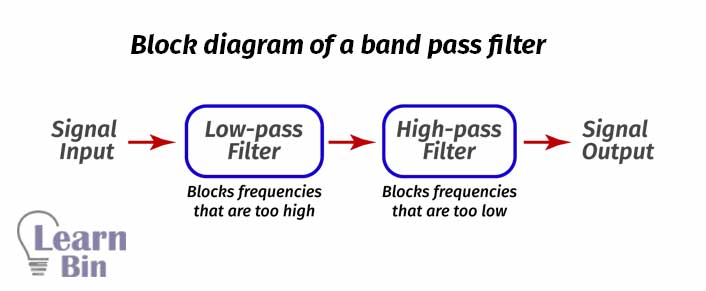Figure 01: Block diagram of a band- pass filter

## Active band pass filter

### Calculation of transfer function of an active band-pass filter

Where, Vin is the input voltage and Vo is the output voltage, the voltage gain of an active band pass filter can be written as follows,

Vin is affected by the impedance given by both the R1 resistor and C1 capacitor. The total impedance can be written as follows,

According to the Kirchhoff law, Vin will be,

Where V+ has been grounded, V- also be zero. Therefore,

Vout is affected by the impedance given by both the R2 resistor and the C2 capacitor. The total impedance can be shown in the following equation,

Output voltage Vo is given by,

Where,

• Vin = Input voltage
• Vo = Output voltage
• R1 = Resistance of the R1 resistor
• R2 = Resistance of the R2 resistor
• C1 = Capacitance of the C1 capacitor
• C2 = Capacitance of the C1 capacitor
• Z1 = Impedance given by R1 resistor and C1 capacitor
• Z2 = Impedance given by R2 resistor and C2 capacitor

Therefore, the voltage gain will be,

Let's take,

The above equation is the transfer function of an active band pass filter.

The difference between the upper critical frequency (fc2) and the lower critical frequency (fc1) is identified as The bandwidth (BW). Upper critical frequency fc2, lower critical frequency fc1, and bandwidth can be calculated as follows.

The frequency that the pass band is centered is known as the center frequency, fo. Center Frequency can be defined as the geometric mean of the critical frequencies. The center frequency is calculated as follows.

## Passive band pass filter

Passive band pass filters can be either RC circuits or RLC circuits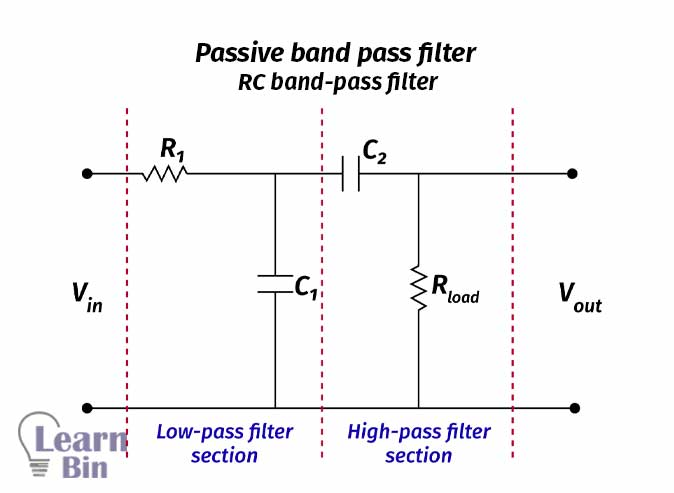Figure 05: Passive band-pass filter | RC band-pass filter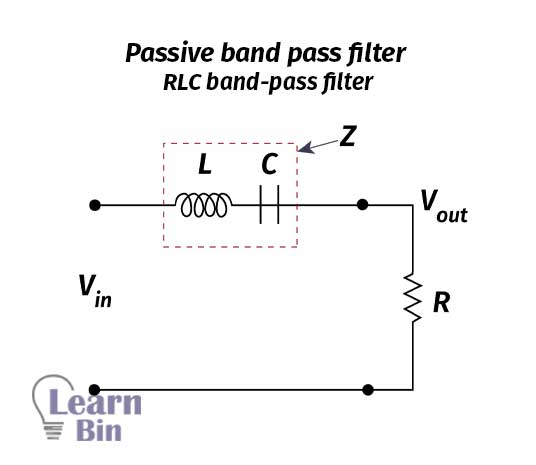Figure 06: Passive band-pass filter | RLC band-pass filter

### Calculation of the transfer function of an RLC band pass filter

Where,

• R = resistance of the R resistor
• ω = Angular frequency of the signal
• C = capacitance of the C capacitor
• L = inductance of the L inductor

For ease of simplification, let’s take the reciprocal of the voltage gain,

To remove the complex parts of the equation, the 4th power of complex parts is taken separately and the 4th root is taken. So, the transfer function is given by,

When ω = ω0 (angular frequency at the center frequency, f0), voltage gain shows the highest value which is 1. At the highest gain,

To fulfill the above requirement, at the center frequency,

For the 3dB cutoff frequency,

By solving the above equation, upper and lower 3dB frequencies can be obtained.

• Lower critical frequency,

• Upper critical frequency,

• Center frequency,

The quality factor (Q) of a band-pass filter can be defined as the ratio of the center frequency to the bandwidth.

If the value of Q (quality factor) is higher, the bandwidth will become narrower. So that shows better selectivity for a given value of fo. (Q>10) as a narrow band or (Q<10) as a wide band.## References and Attributes

### Figures:

The cover image was created using an image by Sergio Stockfleth from Pixabay